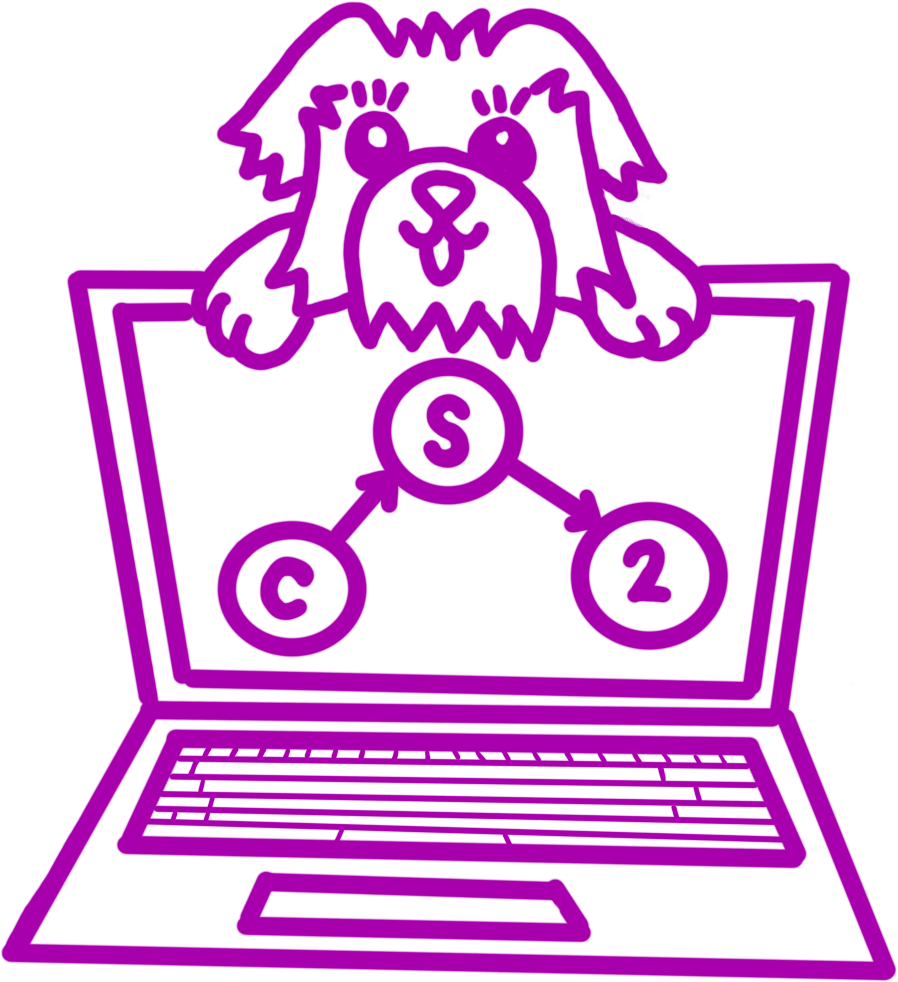##CS 2 (Winter 2022)Lab 06: Grundy's Game

This lab focuses on solving a combinatorial game using recursive functions. It is also an example of a situation where memoization is extremely important.

# Setup

Register for the lab using grinch: https://grinch.caltech.edu/register and clone the repository as explained in the setup instructions.

# Goals and Outcomes

By the end of this lab, you will…

• have more experience with recursive backtracking
• have seen how small changes in a solution result in wildly different solutions and runtimes
• see how combinatorial game theory and programming intersect

# Definitions from Lecture

• A position is the entire state of a game. The definition of a position varies with the game.
• A legal move is a mapping from one position to another position.
• A terminal position is a position where no legal moves are possible.
• A losing position is one in which the previous player to move will win in optimal play. This is also called a $$P$$-position.
• A winning position is one in which the next player to move will win in optimal play. This is also called an $$N$$-position.

We can compute $$P$$ and $$N$$ positions using the following co-recursive definition:

• All terminal positions are $$P$$-positions.
• A position is a $$P$$-position iff all legal moves are $$N$$-positions.
• A position is an $$N$$-position iff there exists a legal move to a $$P$$-position.

# Grundy’s Game

Grundy’s Game is an impartial combinatorial game. It’s very similar to Nim (as discussed in class) in that it’s made up of several “heaps” of identical “tokens”.

In Grundy’s Game…

• A position is a multiset of numbers each indicating the size (number of tokens) in one heap.
• A legal move consists of splitting a single heap into two uneven piles.
• If the position only has heaps of size 0, 1, and 2, it is a terminal position.

## Data Structure Invariants

An invariant is a statement that is true before and after all possible operations on a data structure.

For the GrundyPosition class, we will enforce the following single invariant:

All entries in heapCounts have at least three tokens.

That is, all heaps represented in the position must be active. In a situation where we actually have five heaps, each with one token, and one heap with ten tokens, our representation would omit the five heaps of one token entirely. We’ll see why this is useful later.

## Implementing The GrundyPosition Class

The GrundyPosition class represents a position in Grundy’s Game. The heapCounts field should be a map from height of pile to number of piles of that height in the position. Implement the following methods to simulate a game:

public GrundyPosition(int heapHeight)

Initializes a GrundyPosition which has a single heap with height heapHeight.

public List<GrundyPosition> getMoves()

Returns a List of GrundyPositions representing the legal moves from this position.

### Implementing The Game Solver

Now, use the recursive definition of P and N positions to write a solver for Grundy’s Game.

public boolean isTerminalPosition()

Returns true if and only if this is a terminal position in Grundy’s Game.

public boolean isPPosition()

Returns true if and only if this is a losing position in Grundy’s Game.

public boolean isNPosition()

Returns true if and only if this is a winning position in Grundy’s Game.

### Speeding Up The Computation using Memoization

Add a memoization table (e.g., a HashMap) to memoize recursive calls to isPPosition. Note that there are calls to this method in both isPPostion and isNPosition and both must be saved.

# Getting Checked Off

At the end of every lab, you will need to call over a member of course staff to review your work. Sometimes, we will ask you to think about questions that you’ll review with the person who checks you off. For this lab, please answer the following questions: